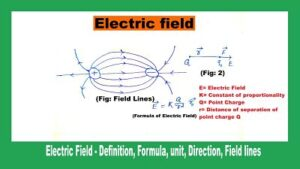# Electric Field – Definition, Formula, unit, Direction, Field lines

## What Is An Electric Field

Definition: Electric field is a region of the space around a charge or system of other charges if another charge is brought in to experience a force. It should be noted that the presence of an electric field around a system of charges even if when there is no charge to realize its effect.

The electric field acts as an intermediary to generate force between two charges and its introduction is indispensable for understanding the interactions between charged bodies. In addition, the area in which the electric field is located has changed or get modified.## The direction of Electric Field

Direction and magnitude are the two important characteristics an electric field. The direction of the electric field at a point is the direction of the force experienced by the positive charge exerted at that point.

So the electric field located at the point close to the positive charge is directed away from it, whereas it directed towards the negative charge.

The electric field is always away from the positive charge and leads to a negative charge. Electric field E is a vector quantity as electric power.

## Electric Field Formula

Mathematically, An electric field is also described as electric force per unit charge. The formula of the electric field is as follows

E = F/Q

Where the letters E, F, and Q represent the following concepts

E = Electric field.

F = Force experienced by charge.

Q = Electric charge

## Electric field intensity / electric field strength

Electric field intensity is also known as electric field strength. The measurement of the strength of electric field strength is called electric field intensity. Electric field intensity and also the electric field is always added vectorially because they are vector quantity.

The electric field strength at a point due to the adjustment of the charge or charges is defined as the force exerted by a unit of positive test charge placed at that particular point.

Mathematically, It can be written as

[latexpage]$$\overrightarrow{\mathrm{E}}=\frac{\overrightarrow{\mathrm{F}}}{\mathrm{q}_{\mathrm{o}}}$$

Whereas,

$\overrightarrow{\mathrm{E}}$ = The electric field intensity.

$\overrightarrow{\mathrm{F}}$ = The force experienced by positive test charge $\mathrm{q}_{\mathrm{o}} .$.

What is a test charge?

It is a positive charge of such a small magnitude. And  it’s presence at a point does not disturb the electric field of that point)

### Unit of electric field intensity

Its unit in SI is Newton per Coulomb and in esu(Electro Static Unit) is dyne per stat Coulomb.

## Concepts of electric Field

The concept of the electric field is used to explain how a charge, or group of charges, affects the field around it. The electric field “E” is similar to “g” which is called acceleration due to gravity but is actually a gravitational field. Learned all about gravity, and how the masses reacts to gravitational forces helps to understand how electric charges react to electric forces.

The electric field for a point charge Q at a distance r is as follows

The electric field from the charge point: E = k Q / r2

Whereas

E= Electric Field

K= Constant of proportionality

Q= Point Charge

r= Distance of separation of point charge Q

If the electric field at a particular point is known, then the net force experienced by charge “q” at that location is as follows:

F = qE

Whereas

F = Net force experience on charges

q = Charge

E= Electric field of that point

If “q” is positive, the force and field will be in one direction.

If “q” is negative, the force will be in the opposite direction of the field.

## Units Of Electric Field

The elementary unit of the electric field is in the International System of Units (SI) it is Newton per Coulomb (N / C).

It is also expressed by the unit of a volt per meter (V / m).

Finally, it can be represented in basic units as kg • m • s − 3 • A − 1 and the dimensional equation is MLT-3I-1.

## What Is Static Electric Field

The electric fields that do not vary with time are called Static electric field (frequency of range 0 Hz).  It is called a constant electric field because Static electric fields are generated by fixed electric charges in space and the field is different from fields and changes over time

For example, electromagnetic fields generated by using AC (alternating current), household appliances, or by mobile phones, etc.

## Dynamic Electric Field

The flow of electric charges through a conductor creates a dynamic electric current and the field created by the motion of charges is called a Dynamic electric field.

## Electric Field Dimensional Formula

The Electric Field is dimensionally represented as [M1 L1 I-1 T-3].

## Energy In The Electric Field

The energy of the electric field is the result of the excitation of the space received by the electric field. It is considered to be the energy that can be supplied with the point charge applied to the field.

## Electric Field Lines

To graphically represent the electric field, Michael Faraday (1791-1867) proposed a representation by means of lines called electric field lines or lines of force.

When drawing these lines you must take into account the following:

• At each point on the electric field lines, the intensity of the electric field (E) is tangent at that point.
• The electric field lines cannot be crossed at any point.
• The electric field lines start from the positive charges and enter the negative charges, hence the positive charges are called sources of the field and the negative charges are called sinks.
• The number of electric field lines leaving or entering the load is proportional to the value of the load.
• More closer the electric field lines, the more intense the electric field will be.
• When the field lines are parallel, the value of the electric field is constant.